# 10-1100/Homework Assignment 4

Jump to: navigation, search

This assignment is due at class time on Tuesday, November 30, 2010.

### Solve the following questions

Problem 1. Prove that a ring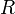$R$ is a PID iff it is a UFD in which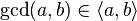$\gcd(a,b)\in\langle a, b\rangle$ for every non-zero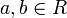$a,b\in R$.

Problem 2. (Selick) In a ring$R$, and element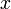$x$ is called "nilpotent" if for some positive$n$,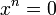$x^n=0$. Let$\eta(R)$ be the set of all nilpotent elements of$R$.

1. Prove that if$R$ is commutative then$\eta(R)$ is an ideal.
2. Give an example of a non-commutative ring$R$ in which$\eta(R)$ is not an ideal.

Problem 3. (comprehensive exam, 2009) Let$A$ be a commutative ring. Show that a polynomial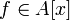$f\in A[x]$ is invertible in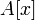$A[x]$ iff its constant term is invertible in$A$ and the rest of its coefficients are nilpotent.

Problem 4. (Lang) Show that the ring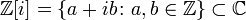${\mathbb Z}[i]=\{a+ib\colon a,b\in{\mathbb Z}\}\subset{\mathbb C}$ is a PID and hence a UFD. What are the units of that ring?

Problem 5. (Dummit and Foote) In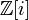${\mathbb Z}[i]$, find the greatest common divisor of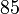$85$ and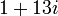$1+13i$, and express it as a linear combination of these two elements.

Problem 6. (Withdrawn, do not submit) Show that the quotient ring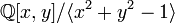${\mathbb Q}[x,y]/\langle x^2+y^2-1\rangle$ is not a UFD.